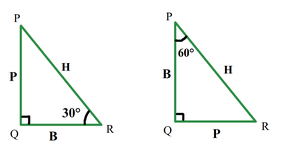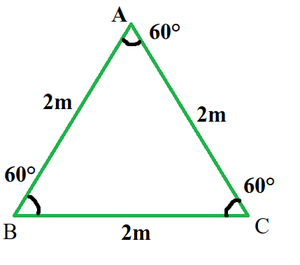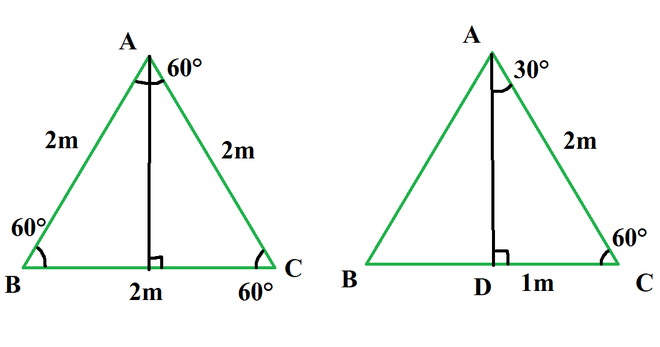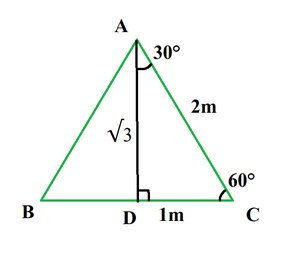# Find the value of tan 30°

• Last Updated : 18 Oct, 2021

Trigonometry is the branch of mathematics that deals with the relationship of sides with angles in a triangle. With trigonometry It is easy to find the heights of big mountains or towers, also in astronomy, it is used to find the distance between stars or planets and is widely used in physics, architecture, and GPS navigation systems.

Trigonometry is based on the principle that “If two triangles have the same set of angles then their sides are in the same ratio”. Side length can be different but side ratios are the same.

Attention reader! All those who say programming isn't for kids, just haven't met the right mentors yet. Join the  Demo Class for First Step to Coding Coursespecifically designed for students of class 8 to 12.

The students will get to learn more about the world of programming in these free classes which will definitely help them in making a wise career choice in the future.

### Trigonometric functions

Trigonometric functions are also called circular functions or trigonometric ratios. The relationship of angles and sides is represented by these trigonometric functions. There are six trigonometric functions Sine, Cosine, Tangent, Cosecant, Secant, Cotangent.

1. Sin = Perpendicular/ Hypotenuse
2. Cos = Base/Hypotenuse
3. Tan = Perpendicular/Base
4. Cot = Base/Perpendicular
5. Sec = Hypotenuse/Base
6. Cosec = Hypotenuse/Perpendicular

Perpendicular, base, and hypotenuse are the three sides of the right-angled triangle. Let’s learn about these terms in detail,

• Perpendicular – The side in front of the angle is perpendicular. In this case, the side in front of 30° is called its perpendicular.
• Base – A base is one of the sides which touches angle, but hypotenuse can never be considered as base.
• What is HypotenusA side opposite to the right angle (90°) is called the hypotenuse, it is the longest side.

Note: Perpendicular and base are not fixed for a triangle. In a triangle, a side is perpendicular for an angle, but the same side is a base for another angle.As shown in the above diagram for the same triangle if, considered angle 30°, perpendicular is the side PQ, but if we consider angle 60° our perpendicular is side QR.

### Find the value of tan 30°.

Solution:

To calculate tan 30°, take ratios of its perpendicular and its base. For which calculate the length of perpendicular and base. To find lengths of sides we take the help of an equilateral triangle.

• Take an equilateral triangle of side length 2m.
• Now from any vertex draw an altitude.
• The altitude drawn divides the equilateral triangle into two right triangles.
• Now we have a length of two sides in the right triangle.
• The third side is calculated by baudhayan theorem or Pythagoras theorem.Altitude in equilateral triangle bisect the angle and the side, So angle DAC is 30° and side DC is 1m.Let the length of side AD is x.

Applying Pythagoras theorem in triangle ADC.

P2 + B2 = H2

x2 + 12 = 22

x2 + 1 = 4

x2 = 3

x = √3Tan30° = 1/√3

Once calculated on all sides one can find other trigonometric ratios also, like:

1. Sin 30° = 1/2
2. Cos 30° = √3/2

### Sample Problems

Question 1: In a right angle triangle, one angle is 30°, and the base for 30° is 3m. Find the length of perpendicular.

Solution:

Given:  Base = 3m

Tan 30 = 1/√3

P/B = 1/√3

P/3 = 1/√3

p = √3

Question 2: In a right triangle hypotenuse is 20cm, and one side is 10√3cm, find the angles of the triangle.

Solution:

Given: H = 20, and B = 10√3

Finding third side using pythagoras theorem.

P2 + B2 = H

P2 + (10√3)2 = 202

p2 + 300 = 400

P2 = 100

p = 10

The third side is 10cm. The ratio of the two sides 10cm and 10√3cm is 1/√3,  So there must be an angle of 30° in triangle Since the triangle is a right angle, so the third angle is

90° – 30° = 60°

The Angles of a triangle are 30°, 60°, 90°.

My Personal Notes arrow_drop_up